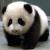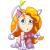## 本人设计的编程语言 热

例如
class Point
{
int X;
int Y;
}

Point.new(int x,int y)
{
Point.X = x;
Point.Y = y;
}

(Point p).print():void
{
Console.Write(p.X+","+p.Y);
}

(Point a)+(Point b):Point
{
return Point.new(a.X+b.Y,a.Y+b.Y);
}

class Point3D()
{
int X;
int Y;
int Z;
}

所有的类都有系统提供的as函数,可以强制转换为某个类
例如:Point3D P3D = Point3D.new(0，0,0);
P3D.as(Point).print();
一个类的字段包含想要转换的类的所有字段才能才能正确转化。
as也可以覆盖.
(string str).as(type Date)
{
return DateTime.Parse(str);
}
-------------------------------------------------------------------------------------------------

0class object
{
}
因为object没有任何字段,所以任何类都可以转化成object

自由式函数:
(Human A) user (Computer C) print (File F);//英语不好,见笑.意思是人A用电脑C打印文件F；

Select from (List<Human> list) where (string where) order by (string propterty):List<Human> list

0